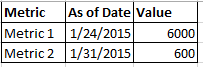# QlikView App Dev

Discussion Board for collaboration related to QlikView App Development.

Announcements
Customer & Partners, DEC. 9, 11 AM ET: Qlik Product & Strategy Roadmap Session: Data Analytics REGISTER NOW
cancel
Showing results for
Did you mean:Partner

## Find a value when 2 dates match

I'm having trouble figuring out the proper syntax for a formula and looking for some help.  In my data model, I have data that looks similar to below.  I have multiple metrics each one having different amounts of history (CLNDR_DT column) as well as different timing of when the data was last refreshed (AS_OF_DATE column.

What I am trying to do is display this data in a straight table but I want 1 line per metric and the value to be the Value where the CLNDR_DT = AS_OF_DATE as represented in the green rows below.So the straight table would look something like this:I have tried the following formulas and I can't get them to do what I am wanting.  They seem to be looking at the max AS_OF_DATE for all metrics and not keeping it metric specific.

sum({<CLNDR_DT = {AS_OF_DATE}>}  Value)

sum({<CLNDR_DT = {Max(AS_OF_DATE)}>}  Value)

sum({<CLNDR_DT = {"\$(=Max(AS_OF_DATE))"}>}  Value)

I hope this was clear enough.  What am I missing?

1 Solution

Accepted SolutionsPartner
Author

Thank you everyone.  I actually went in a slightly different direction.  I'm curious what you think.

I actually made a calculated dimension like the following:

=if(CLNDR_DT = AS_OF_DATE, Metric)

Seems to be working for me.

6 RepliesChampion III

Try using if():

sum(if(floor(CLNDR_DT) = floor(AS_OF_DATE), Value))

(I added floor() here in case the dates are actually timestamps)Partner

- Value / (not CLNDR_DR-AS_OF_DATE)MVP

Playing off what others have suggested.

=sum(Value * (CLNDR_DT = AS_OF_DATE) * -1)

or

=sum({<CLNDR_DT={"=CLNDR_DT=AS_OF_DATE"}>}Value)

If it's always the highest CLNDR_DT rows you want for each Metric:

=FirstSortedValue(Value, - CLNDR_DT)

-Rob

http://robwunderlich.comPartner
Author

Thank you everyone.  I actually went in a slightly different direction.  I'm curious what you think.

I actually made a calculated dimension like the following:

=if(CLNDR_DT = AS_OF_DATE, Metric)

Seems to be working for me.MVP

Interesting. I would not have thought of that one. The only downside I can see is if you want to add another expression that processes multiple rows, like Avg(Value). But it actually makes it easier to add other dimension values from the same row. So that's a plus.